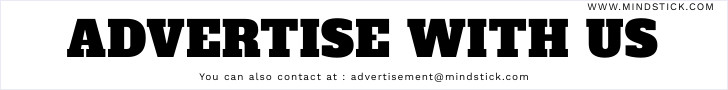# What is the difference between == and === ?

Posted by  Vijay Shukla
2891  View(s)
Ratings:
Rate this:
1.##### What is the difference between == and === ?

The 3 equal signs mean "equality without type coercion". Using the triple equals, the values must be equal in type as well.

== is equal to
=== is exactly equal to (value and type)
0==false // true
0===false // false, because they are of a different type
1=="1" // true, auto type coercion
1==="1" // false, because they are of a different type